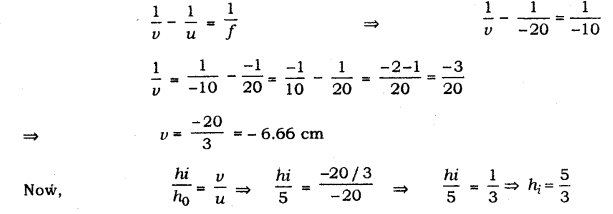# An object of height 5 cm is placed perpendicular to the principal axis of a concave lens of focal length 10 cm

An object of height 5 cm is placed perpendicular to the principal axis of a concave lens of focal length 10 cm. If the distance of the object from the optical centre of the lens is 20 cm, determine the position, nature and size of the image formed using the lens formula.

Focal length of the concave lens, f = - 10 cm
Object distance, u = - 20 cm
Let the image distance be v.
Object size, h0 = 5 cm
According to the lens formula,Thus, the image formed is between lens and focal length, the image is diminished, virtual and Erect